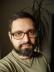Diff-K

You are given an array @N of positive integers (sorted) and another non negative integer \$k. Write a script to find if there exists 2 indices \$i and \$j such that \$A[\$i] - \$A[\$j] == \$k and \$i != \$j. It should print the pairs of indices, if any such pairs exist.

Example:

@N = (2, 7, 9);
\$k = 2;

Output: 2, 1

I totally ignored the fact that the input array is sorted. My solution works for any input array, but it’s still rather efficient.

The basic trick is that we don’t have to compute \$A[\$i] - \$A[\$j] for each combination or \$i and \$j. We know \$k from the very beginning, so we can just iterate the array for the first time to store it in a hash, and iterate it for the second time to check the hash whether the corresponding number exists in the array.

#!/usr/bin/perl
use warnings;
use strict;
use feature qw{ say };

my @N = (2, 7, 9);
my \$k = 2;

my %in_array;
@in_array{ @N } = 0 .. \$#N;

for (@N) {
say join ', ', @in_array{ \$k + \$_, \$_ }
if exists \$in_array{ \$k + \$_ };
}

It works for the sample input, but it has a problem: It doesn’t work if a number is repeated in the array (because hash keys are unique). To fix it, we need to use a hash of arrays instead of a plain hash, and for each number store all the indices it appears at.

#!/usr/bin/perl
use warnings;
use strict;
use feature qw{ say };

my @N = (2, 7, 9, 2, 7, 4);
my \$k = 2;

my %in_array;
push @{ \$in_array{ \$N[\$_] } }, \$_ for 0 .. \$#N;

for my \$n (0 .. \$#N) {
if (my \$found = \$in_array{ \$N[\$n] + \$k }) {
say "\$_, \$n" for @\$found;
}
}

The output lists all the possible pairs:

5, 0
2, 1
5, 3
2, 4

Path Sum

You are given a binary tree and a sum, write a script to find if the tree has a path such that adding up all the values along the path equals the given sum. Only complete paths (from root to leaf node) may be considered for a sum.

Example

Given the below binary tree and sum = 22,

5
/ \
4   8
/   / \
11  13  9
/  \      \
7    2      1

For the given binary tree, the partial path sum 5 → 8 → 9 = 22 is not valid. The script should return the path 5 → 4 → 11 → 2 whose sum is 22.

So we are only interested in leaf nodes. Note that for each node, the sum corresponds to the sum of its parent plus the value of the node itself. We can process the tree in the depth-first order (using recursion), computing the sum for each node. If we arrive at a leaf node, we remember the sum. We also need to remember the path, but it’s similar: the path of a node is its parent’s path joined with the node itself.

At the beginning, I flirted with the idea of implementing a parser of the input tree, but I realised the format hadn’t been precisely specified, so I decided to postpone it. In hindsight, it was a good decision, otherwise I’d be bored this week.

#!/usr/bin/perl
use warnings;
use strict;
use feature qw{ say };

use Syntax::Construct qw{ // };

sub fill_sum {
_fill_sum(\$_, 0, [], my \$path_sums = {});
return \$path_sums
}

sub _fill_sum {
my (\$tree, \$parent_sum, \$parent_path, \$path_sums) = @_;
\$tree->{s} = \$tree->{v} + (\$parent_sum // 0);
\$tree->{p} = [ @\$parent_path, \$tree->{v} ];
if (\$tree->{ch}) {
_fill_sum(\$_, \$tree->{s}, \$tree->{p}, \$path_sums)
for @{ \$tree->{ch} };
} else {
push @{ \$path_sums->{ \$tree->{s} } }, \$tree->{p};
}
}

my \$tree = {
v => 5, ch => [
{ v => 4, ch => [
{ v => 11, ch => [
{ v => 7 },
{ v => 2 }
] }
] },
{ v => 8, ch => [
{ v => 13 },
{ v => 9, ch => [
{ v => 1 }
] }
] }
] };
my \$sum = 22;

my \$path_sums = fill_sum(\$tree);
say join '->', @\$_ for @{ \$path_sums->{\$sum} // [] };

As you can see, v stands for “value”, ch is for “children”, p means the “path”, and s represents the “sum”.I blog about Perl.